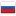Prefix sums k times

Revision en1, by Skeef79, 2021-10-26 17:45:42

Hello codeforces!

I am wondering is there a way to caculate prefix sums $k$ times modulo $p$ fast, especially when we can't use matrix multiplication?

By calculating prefix sums $k$ times I mean this piece of code:

for(int it = 0; it < k; it++) {
for(int i = 1; i < n; i++)
pref[i]+= pref[i-1];
}


So if we start with array: $1, 0, 0, 0$ we will get:

1. $1, 1, 1, 1$;

2. $1, 2, 3, 4$;

3. $1, 3, 6, 10$;

4. $1, 4, 10, 20$; ...

#### History

RevisionsRev. Lang. By When Δ Comment
en1Skeef79 2021-10-26 17:45:42 500 Initial revision for English translation
ru1Skeef79 2021-10-26 17:41:50 500 Первая редакция (опубликовано)